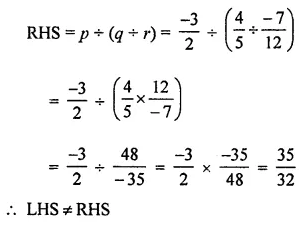# ML Aggarwal Class 8 Solutions for ICSE Maths Chapter 1 Rational Numbers Ex 1.4

## ML Aggarwal Class 8 Solutions for ICSE Maths Chapter 1 Rational Numbers Ex 1.4

Question 1.
Find the value of the following:Solution: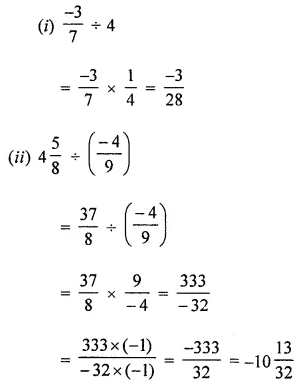Question 2.
State whether the following statements are true or false:
(i) $$\frac { -9 }{ 13 }$$ ÷ $$\frac { 2 }{ 7 }$$ is a rational number.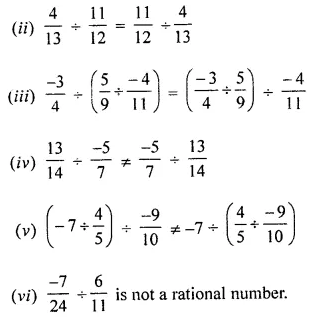Solution: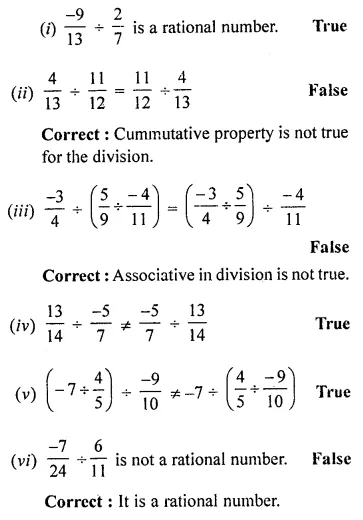Question 3.
The product of two rational numbers is $$\frac { -11 }{ 12 }$$. If one of them is 2$$\frac { 4 }{ 9 }$$, find the other.
Solution: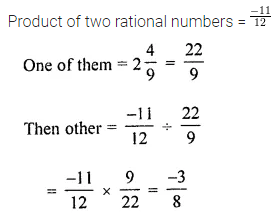Question 4.
By what rational number should $$\frac { -7 }{ 12 }$$ be multiplied to get the product as $$\frac { 5 }{ 14 }$$ ?
Solution:Question 5.
By what rational number should -3 is divided to get $$\frac { -9 }{ 13 }$$?
Solution:Question 6.
Divide the sum of $$\frac { -13 }{ 8 }$$ and $$\frac { 5 }{ 12 }$$ by their difference.
Solution: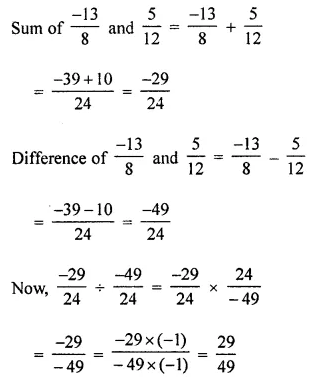Question 7.
Divide the sum of $$\frac { 8 }{ 3 }$$ and $$\frac { 4 }{ 7 }$$ by the product of $$\frac { -3 }{ 7 }$$ and $$\frac { 14 }{ 9 }$$.
Solution: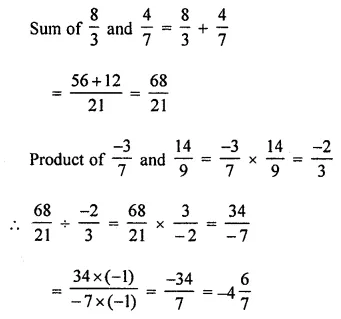Question 8.
If p = $$\frac { -3 }{ 2 }$$, q = $$\frac { 4 }{ 5 }$$ and r = $$\frac { -7 }{ 12 }$$, then verify that (p ÷ q) ÷ r ≠ p ÷ (q ÷ r).
Solution: